## Surface Area of a Right Rectangular Prism

This post about finding the surface area of a right rectangular prism is part of a series of posts to help you prepare for the Advanced Algebra and Functions part of the Accuplacer test.

## Question

What is the surface area of the right rectangular prism shown? Its length is 6, its width is 5, and its height is 4.Right rectangular prism for the example

## Solution

### Sidebar: Surface Area of a Right Rectangular Prism

A right rectangular prism is the shape of a box with a rectangle for a bottom, with sides perpendicular to its bottom, and with a flat top parallel to its bottom. It’s your typical box.

A right rectangular prism’s surface area is the sum of the areas of all its faces: the top, the bottom, and the four sides. The area of each side is length times width.

This box has six sides, three pairs of congruent sides.

• Let’s start with the side that stares you in the face on the diagram. It measures 4 x 5, so its area is 20. There is an identical piece in the back with the same area; the area of the two together is 2 times 20 = 40.
• Now consider the sides on the left and right. (In the diagram, you can’t see the side on the left.) Each of those sides measures 6 x 4, so each one’s area is 24. The area of the two together is 2 times 24 = 48.
• The top has a length of 6 and a width of 5, so its area is 6 X 5 = 30. The bottom has the same area, so the area of the two together is 2 times 30 = 60.

Then the surface area of the whole prism is

And the answer is 148 square units.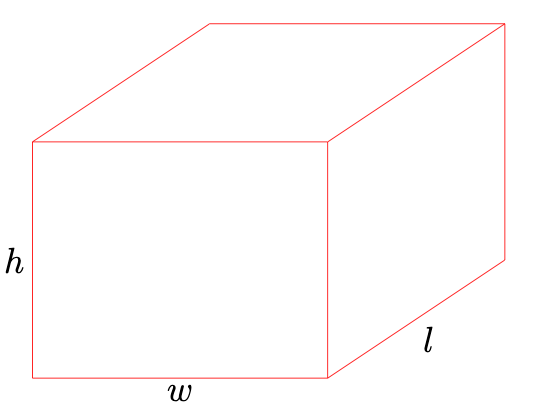Right rectangular prism for formula

### In general

The formula for the surface area of a right rectangular prism — a box — is

where SA surface area and h, w, and l are as shown on the box on the right.

This question is similar to question number 3 in the sample questions for the Accuplacer Advanced Algebra and Functions test.

## Congruent Triangles

This post about congruent triangles is part of a series of posts to help you prepare for the Advanced Algebra and Functions part of the Accuplacer test.

## Question

Angle A in triangle ABC is congruent to angle D in triangle DEF. Which of the statements below, if it’s true, proves that triangles ABC and DEF are congruent?

A.  and

B.   and

C.   and

D.   andTriangle DEF.Triangle ABC.

## Solution

Two triangles are congruent if one of these things is true:

• every side of one is congruent to a side of the other (SSS);
• two sides and the angle between them in one are congruent to two sides and the angle between them in the other (SAS); or
• two angles and any side in one are congruent to two angles and any side of the other (ASA or AAS).

Let’s go through the answer choices to see if one of them meets one of those criteria.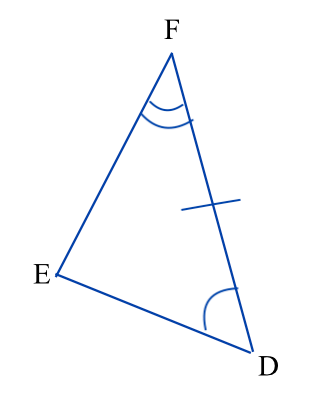Triangle DEF

A.  and .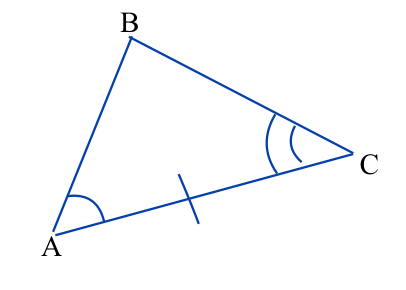Triangle ABC

That’s  two  angles and the side between them in one triangle being congruent to the corresponding parts in the other triangle. That’s enough to meet the first criterion above, so it looks like that’s the answer. But let’s check the others.

B.   and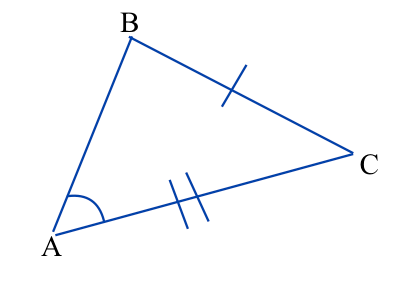Triangle ABC.

That’s two sides and an angle, but not the angle between them, so it does not do the job.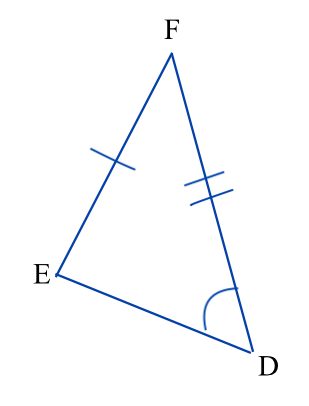Triangle DEF

C.   and .  Same problem as answer choice B: Two sides and one angle of one are congruent to two sides and one angle of the other, but the angle is not the one between the two sides, so that information is not enough for a proof.Triangle DEFTriangle ABC

D.  and . This gives you only congruent angles. Every proof of congruent triangles requires at least one side.Triangle ABC